﻿ Mathematical Formulas And Calculations. Math Education Concept. Stock Image - Image of extraction, economy: 35809623

# Mathematical formulas and calculations. Math education concept.

## Mathematical formulas and calculations in university textbook. Math education concept.

Photo Taken On: December 06th, 2013

Designers also selected these stock photos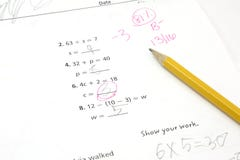5th Grade Math TestTeenager girl relax home - happy listen to music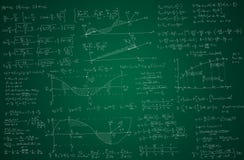Math backgroundLight flower background - spring design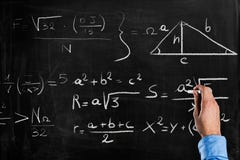Math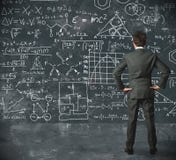Businessman and problems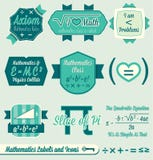Vector Set: Vintage Math Labels and Icons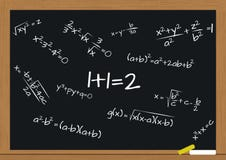Formula mathDizzy person seeing stars
More similar stock images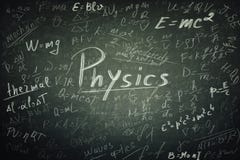Physics formulas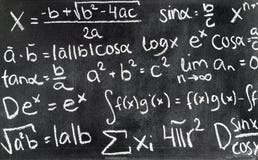Handwritten mathematical formulas on blackboard written.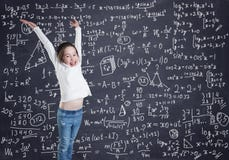A little girl in front of the blackboard with formulas and calculationsSmart scientist with scientific backgroundDoing homework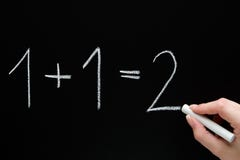Basic Calculations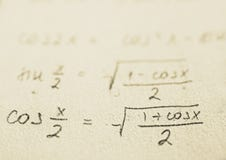School - Math formulas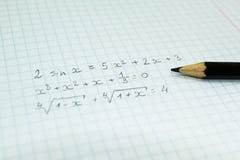Mathematical formulas in a notebook for lectures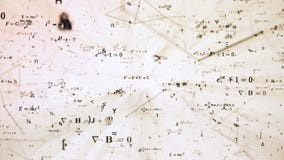Digital futuristic illustration with math, physics formulas in mesh network grid
More stock photos from Mateusz Żogała's portfolio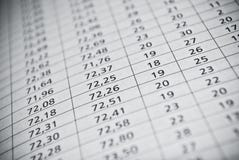Mathematical education concept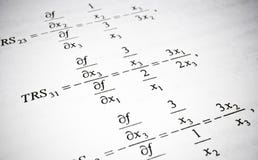Mathematical formulas and calculations. Math education concept.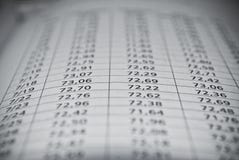Mathematical education concept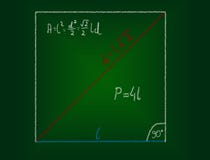Mathematical education concept of chalkboard and drawing.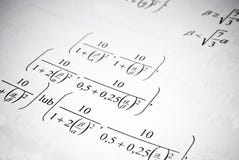Mathematical formulas and calculations. Math education concept.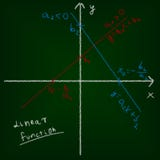Mathematical education concept of chalkboard and drawing.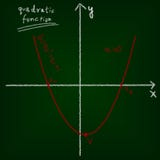Mathematical education concept of chalkboard and drawing.
Related categories
Extended licenses